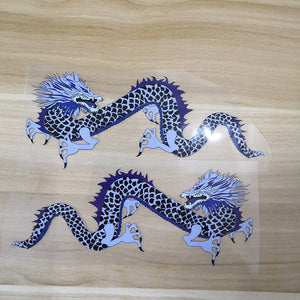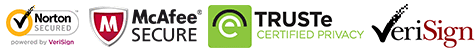FedEx will stop during Oct 1-8
FedEx will stop during Oct 1-8# Blue Dragon Patches for DIY / Custom Air Force 1 Dragon

Regular price \$19.80 \$9.90 Save \$9.90

ONLY 199906 LEFT

 Hours   Minutes   Seconds

Sale Ends Once The Timer Hits Zero!

ONLY 199906 LEFT

Get these easy use iron on blue dragon patches to DIY to create your own dragon shoes. Best size for DIY or custom Air Force 1 & Vans.

<<<<<<<<<<<<<<<<<<<<<<<<<<<<<<<<<<<<<<<<<<<<<<<<<<<<<<<

### Why use these dragon patches:

1. Perfect sizes for sneakers: AF1, Vans, Converse or other shoes.

2. Also can be used on cloth, socks. hoodies, jeans, tshirt....

3. Durable, waterproof and flexible.

4. Easy use, just need to iron on top.

5. Get these blue dragon patches to DIY, save lot's money to buy dragon shoes.

<<<<<<<<<<<<<<<<<<<<<<<<<<<<<<<<<<<<<<<<<<<<<<<<<<<<<

The real one is beautiful than the picture, the picture had changed a little due to the displayer.

The set includes 2 blue dragons

The shoes are not included in the set.

<<<<<<<<<<<<<<<<<<<<<<<<<<<<<<<<<<<<<<<<<<<<<<<<<<<<<<<

Size:

width 17 cm, 6.7inches

Height: 7 cm, 2.76 inches

<<<<<<<<<<<<<<<<<<<<<<<<<<<<<<<<<<<<<<<<<<<<<<<<<<<<<<<

### How to use:

1. Position the dragon patches on the shoes with tape

2. Iron on the top of the dragon, please keep temperature 130-150℃

3. peel the vinyl, the dragon patches will stick on the shoes.

4. If use normal iron, please turn off the steam, keep the temperature above, use the tip and be careful and patient, for it's heavy to control, better use the mini iron.

<<<<<<<<<<<<<<<<<<<<<<<<<<<<<<<<<<<<<<<<<<<<<<<<<<<<<<<

### Free shipping

people are currently looking at this product

Estimated delivery time 7-15 days

Guaranteed Safe Checkout